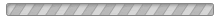# Electrical Power System

## Jadwal Pelatihan Electrical Power System

TanggalKota

DESCRIPTION

This course is an introduction to the field of electric power systems. Material covered in the subject includes: Fundamentals of energy conversion system. Modeling of main power network components such as power transmission lines, transformers and synchronous machines. Basic description of their behavior using appropriate representations. Simplification of problems using transformation techniques. Fundamentals of network analysis, power system representation, power load flow. Review of symmetrical components and short circuit analysis.

OBJECTIVE

• Describe of the common methods of converting energy to electric power.
• Descrive the classical power system structure.
• Perform steady-state analysis for a balanced three-phase power system.
• Represent elements of a power system including generators, transmission lines, and transformers.
• Understand the functioning of a synchronous machine and represent it with simple models.
• Construct a composite system by the interconnection of the elements of a power system.
• Analyze multi-node power systems using an admittance matrix or impedance matrix representation of the power system.
• Factor the admittance matrix to obtain a solution of the network voltages.
• Generate the elements of the impedance matrix from the elements of the admittance matrix without a matrix inversion.
• Understand the formulation of the power flow problem, and have the ability to cast any given system in this framework.
• Solve power flow problems by application of the Newton method.
• Design a transmission addition to a power system using tools and methods from the course.
• Formulate a mathematical model for analyzing faults on power systems.

CONTENT

1. Introduction to Electrical Energy System

• Sources of Energy
• Electromechanical Energy Conversion
• Environmental Impact of Electricity Generation and Transmission
• The Generation Subsystem
• The Transmission Subsystem
• The Distribution Subsystem
• Power Industry Structure

2. Introduction to Polyphase Networks Analysis

• Balanced Three-Phase Circuits :
• Delta Connection
• Wye Connection
• Power Calculations in Balanced Three-Phase Circuits
• Per-Phase Analysis

3. Transformers and Per-Unit Systems

• The Ideal Transformer
• Three-Phase Transformer
• An Actual Transformer
• Introduction to Per-Unit Systems

4. Electric Power Generation and Transmission Systems

• Electric Power Generation
• Electric Power Transmission
• Transmission Line Components
• Complex Power Transmission

• Power Flow
• The Power Flow Problem
• Newton-Raphson Iteration
• Application to Power Flow Equations

TRAINING METHOD

Presentation

Discussion

Practice

Case Study

Evaluation

FACILITIES

Training Kit

Flash disk

Handout

Certificate

Lunch + 2 X Coffee Break

Souvenir

Pickup Paricipant

TRAINING FEE for Electrical Power System

Rp7.500.000,-/Participant/Non Residential

#### Data Materi Training

Topik Training : Electrical Power System
*Jumlah Peserta Estimasi Jumlah Peserta yang di ajukan
*Nama Peserta Yang Didaftarkan

#### Personal Data

*Nama
*Jabatan Jabatan/Divisi/Departement
*Nama Perusahaan
*Alamat Perusahaan
*Email Perusahaan
*Email Alternatif eg: gmail, yahoo, hotmail
*Telepon Kantor
Ekstensi
*Handphone
* Harus di isi### Related Articles

#### Check Also

Close
*Semua Silabus dan jadwal training yang ada di Training SDM adalah milik dari masing-masing vendor penyelenggara training.
Close